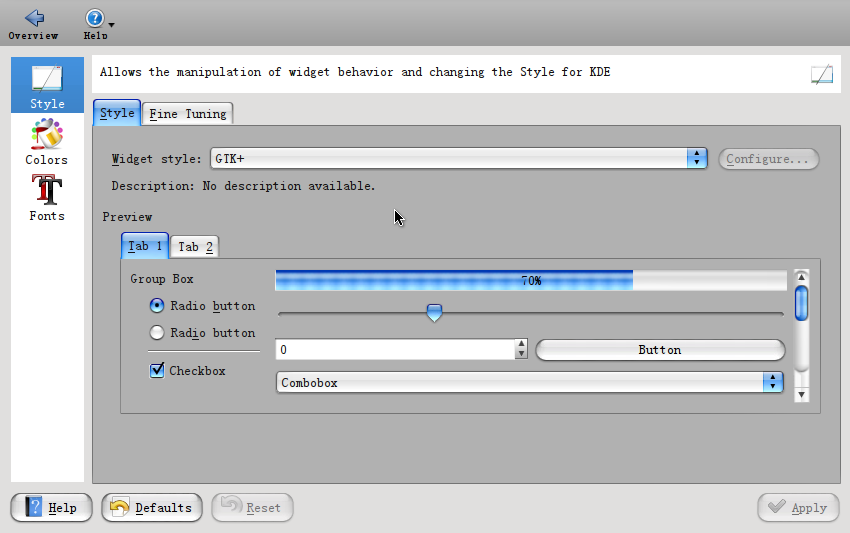#include <stdio.h>
#include <math.h>

long gcd(long a,long b) {
return b ? gcd(b, a % b) : a;
}

long lcm(long a, long b) {
return a / gcd(a, b) * b;
}

int main(int argc, char *argv[]) {
long a, b;
long m;
int sum;

while(scanf("%ld%ld", &a, &b) != EOF) {
if (a == b) {
sum = 1;
}
else {
m = a * b;

sum = 0;
int i;

for (i = 1; i < sqrt(m); ++i) {
if (((m % i) == 0) && (gcd(i, m/i) == a)) {
sum += 1;
}
}

sum *= 2;
}
printf ("%d\n",sum);
}
return 0;
}

sudo emerge -av gnome-lightsudo emerge -av systemsettings